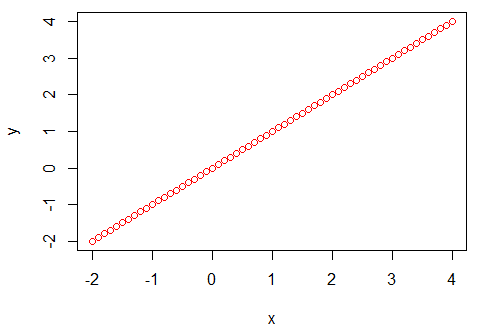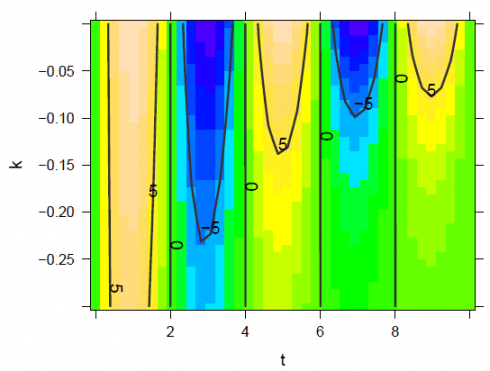# Articles by Kristian Larsen

### Integration in R

July 5, 2019 |

Are you interested in guest posting? Publish at DataScience+ via your editor (i.e., RStudio). Category Basic Statistics Tags Linear Regression R Programming Tips & Tricks Integration is the process of evaluating integrals. It is one of the two central ideas of calculus and is the inverse of the other central ...### Calculus in R

June 27, 2019 |

Are you interested in guest posting? Publish at DataScience+ via your editor (i.e., RStudio). Category Basic Statistics Tags Data Management Linear Regression R Programming Tips & Tricks In this article you learn how to do calculus with R. Introdutcion to Calculus Calculus is a branch of mathematics that involves the ...